Download the eSaral app and start learning from Kota's top IITians and doctors.

# NCERT Solutions for Class 9 Science Chapter 3 - Atoms and Molecules - eSaral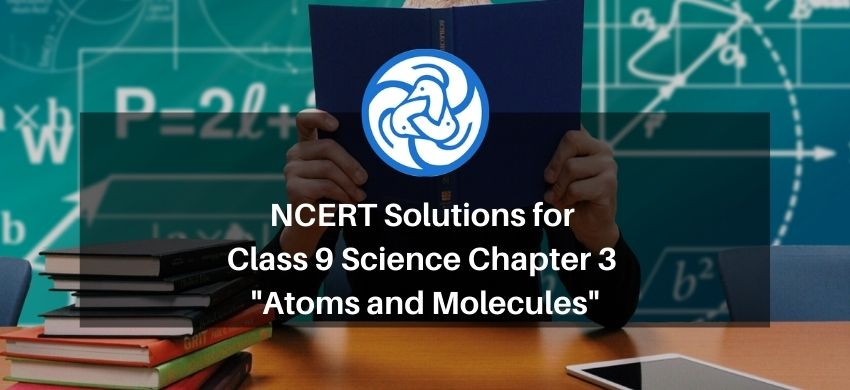Hey, are you a class 9 student and looking for ways to download NCERT Solutions for Class 9 Science Chapter 3 "Atoms and Molecules"? If yes. Then you are at the right place.

In this article, we have listed NCERT solutions for class 9 Science Chemistry Chapter 3 in PDF that is prepared by Kota’s top expert Chemistry Faculties by keeping Simplicity in mind.

If you want to learn and understand class 9 Science chapter 3 "Atoms and Molecules" in an easy way then you can use these solutions PDF.

NCERT Solutions helps students to Practice important concepts of subjects easily. Class 9 Science solutions provide detailed explanations of all the NCERT exercise questions that students can use to clear their doubts instantly.

If you want to score high in your class 9 Science Exam then it is very important for you to have a good knowledge of all the important topics, so to learn and practice those topics you can use eSaral NCERT Solutions.

So, without wasting more time Let’s start

### Download The PDF of NCERT Solutions for Class 9 Science Chapter 3 "Atoms and Molecules"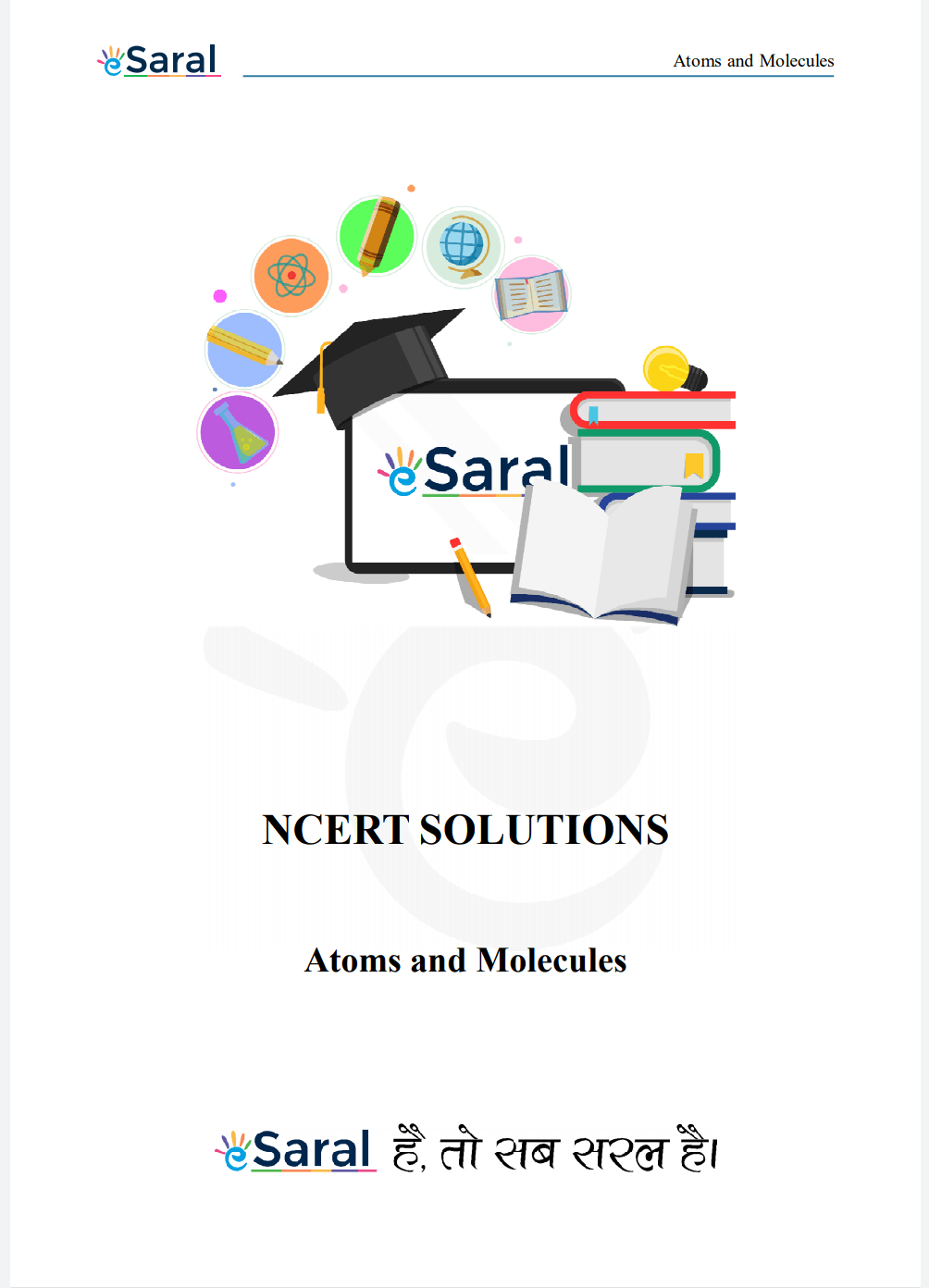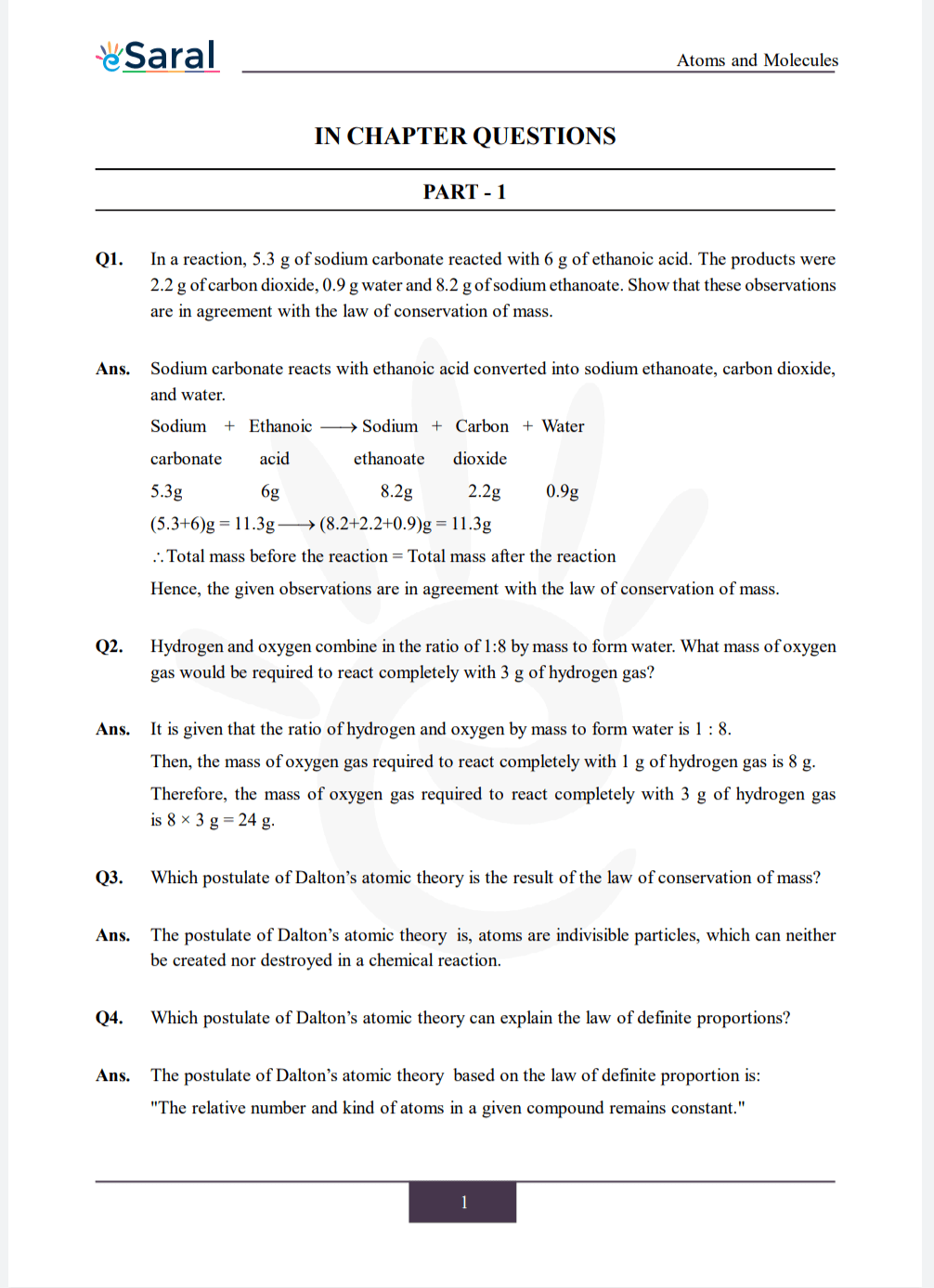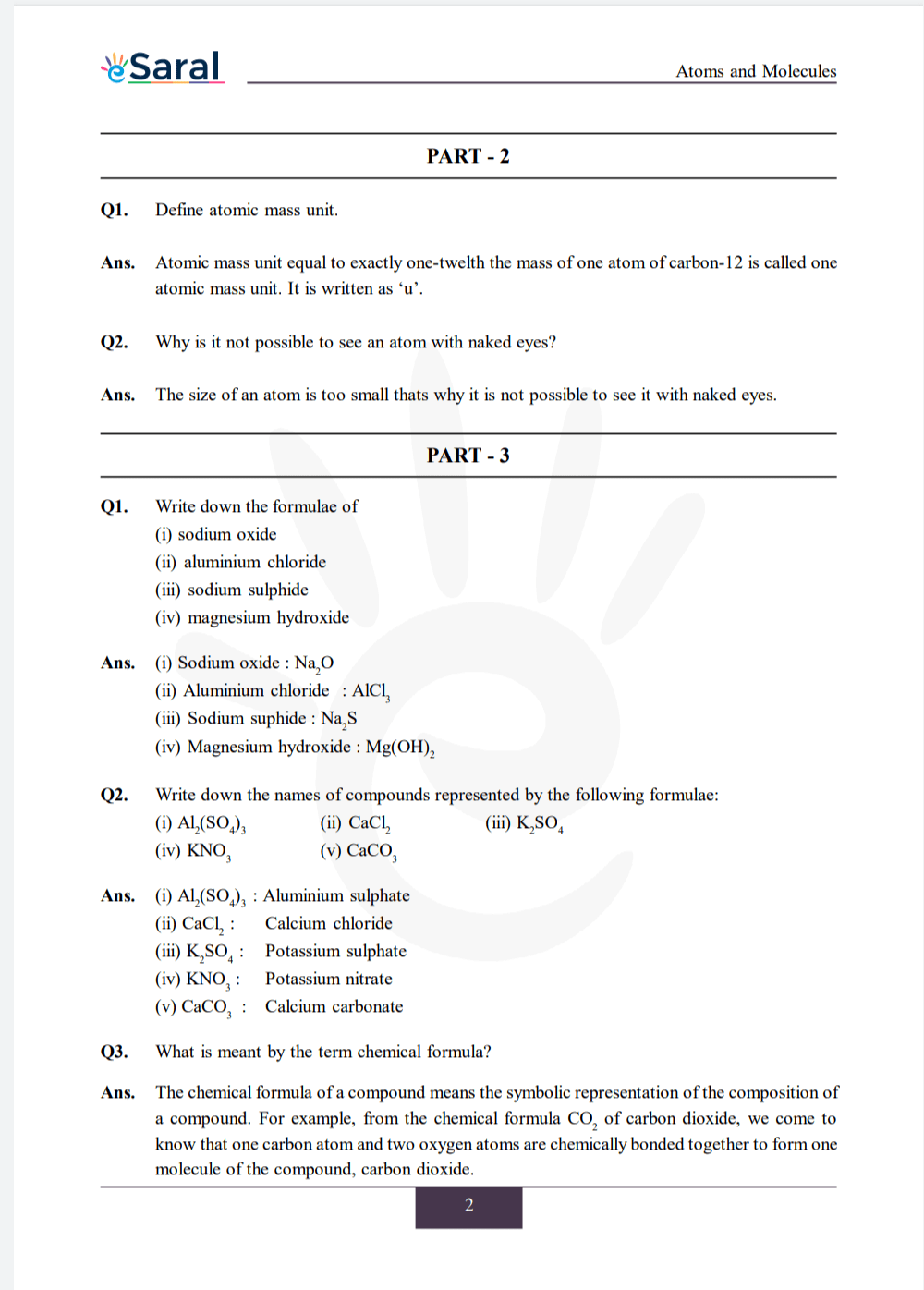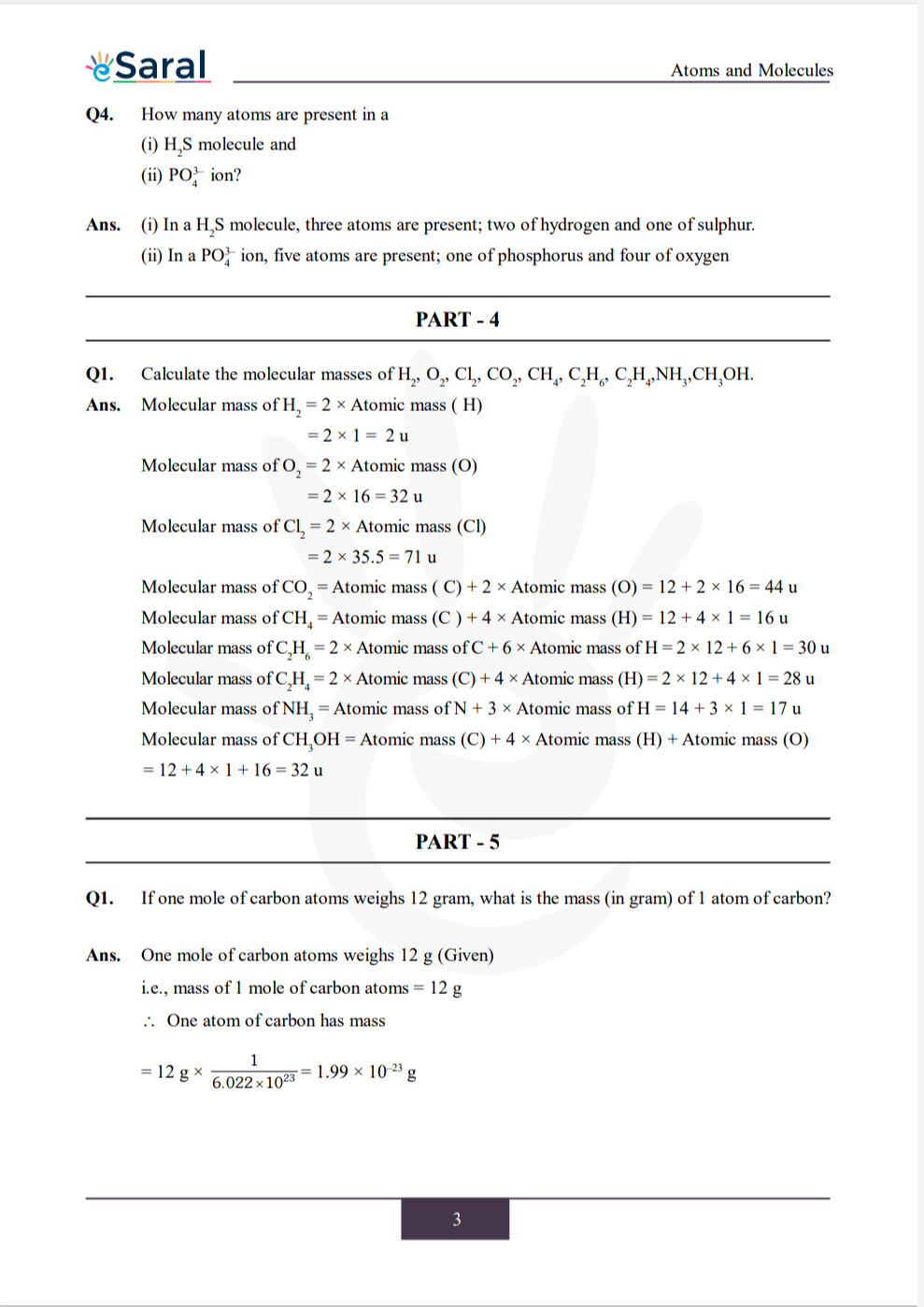Download The PDF of NCERT Solutions for Class 9 Science Chapter 3 "Atoms and Molecules"

### Important topics of class 9 Science Chapter 3

• Laws of Chemical Combinations
• What is an Atom
• What is a Molecule
• Molecular Mass and Mole Concept
• Writing Chemical Formulae
• Atoms and Molecules

#### All Questions of Chapter 3

Once you complete the chapter 3 then you can revise it by solving following questions.

Q1. In a reaction, 5.3 g of sodium carbonate reacted with 6 g of ethanoic acid. The products were 2.2 g of carbon dioxide, 0.9 g water and 8.2 g of sodium ethanoate. Show that these observations are in agreement with the law of conservation of mass.

Q2. Hydrogen and oxygen combine in the ratio of 1:8 by mass to form water. What mass of oxygen gas would be required to react completely with 3 g of hydrogen gas?

Q3. Which postulate of Dalton’s atomic theory is the result of the law of conservation of mass?

Q4. Which postulate of Dalton’s atomic theory can explain the law of definite proportions?

##### Part 2

Q1. Define atomic mass unit.

Q2. Why is it not possible to see an atom with naked eyes?

##### Part 3

Q1. Write down the formulae of
(i) sodium oxide
(ii) aluminium chloride
(iii) sodium sulphide
(iv) magnesium hydroxide

Q2. Write down the names of compounds represented by the following formulae:
(i) $\mathrm{Al}_{2}\left(\mathrm{SO}_{4}\right)_{3}$
(ii) $\mathrm{CaCl}_{2}$
(iii) $\mathrm{K}_{2} \mathrm{SO}_{4}: \quad$ Potassium sulphate
(iv) $\mathrm{KNO}_{3}: \quad$ Potassium nitrate
(v) $\mathrm{CaCO}_{3}: \quad$ Calcium carbonate

Q3. What is meant by the term chemical formula?

Q4. How many atoms are present in a
(i) $\mathrm{H}_{2} \mathrm{~S}$ molecule and
(ii) $\mathrm{PO}_{4}^{3-}$ ion?

##### Part 4

Q1. Calculate the molecular masses of $\mathrm{H}_{2}, \mathrm{O}_{2}, \mathrm{Cl}_{2}, \mathrm{CO}_{2}, \mathrm{CH}_{4}, \mathrm{C}_{2} \mathrm{H}_{6}, \mathrm{C}_{2} \mathrm{H}_{4}, \mathrm{NH}_{3}, \mathrm{CH}_{3} \mathrm{OH}$.

##### Part 5

Q1. If one mole of carbon atoms weighs 12 gram, what is the mass (in gram) of 1 atom of carbon?

Q2. Which has more number of atoms, 100 grams of sodium or 100 grams of iron (given, atomic mass of $\mathrm{Na}=23 \mathrm{u}, \mathrm{Fe}=56 \mathrm{u}$ ) ?

##### Exercise

Q1. A 0.24 g sample of compound of oxygen and boron was found by analysis to contain 0.096 g of boron and 0.144 g of oxygen. Calculate the percentage composition of the compound by weight.

Q2. When 3.0 g of carbon is burnt in 8.00 g oxygen, 11.00 g of carbon dioxide is produced. What mass of carbon dioxide will be formed when 3.00 g of carbon is burnt in 50.00 g of oxygen? Which law of chemical combinations will govern your answer?

Q3. What are polyatomic ions? Give examples?

Q4. Write the chemical formulae of the following:
(a) Magnesium chloride
(b) Calcium oxide
(c) Copper nitrate
(d) Aluminium chloride
(e) Calcium carbonate

Q5. Give the names of the elements present in the following compounds:

(a) Quick lime
(b) Hydrogen bromide
(c) Baking powder
(d) Potassium sulphate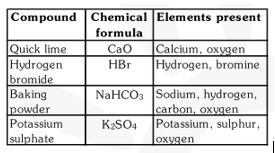Q6. Calculate the molar mass of the following substances
(a) Ethyne, $\mathrm{C}_{2} \mathrm{H}_{2}$
(b) Sulphur molecule, $\mathrm{S}_{8}$
(c) Phosphorus molecule, $\mathrm{P}_{4}$ (atomic mass of phosphorus $=31$ )
(d) Hydrochloric acid, $\mathrm{HCl}$
(e) Nitric acid, $\mathrm{HNO}_{3}$

Q7. What is the mass of
(a) 1 mole of nitrogen atoms?
(b) 4 moles of aluminium atoms (Atomic mass of aluminium $=27$ )?
(c) 10 moles of sodium sulphite $\left(\mathrm{Na}_{2} \mathrm{SO}_{3}\right)$ ?

Q8. Convert into mole.
(a) 12 g of oxygen gas
(b) 20 g of water
(c) 22 g of carbon dioxide

Q9. What is the mass of :
(a) 0.2 mole of oxygen atoms ?
(b) 0.5 mole of water molecules ?

Q10. Calculate the number of molecules of sulphur $\left(\mathrm{S}_{8}\right)$ present in $16 \mathrm{~g}$ of solid sulphur.

Q11. Calculate the number of aluminium ions present in $0.051 \mathrm{~g}$ of aluminium oxide.(Hint: The mass of an ion is the same as that of an atom of the same element. Atomic mass of $\mathrm{Al}=27 \mathrm{u}$ )

Science Class 9 Complete Revision Videos